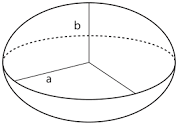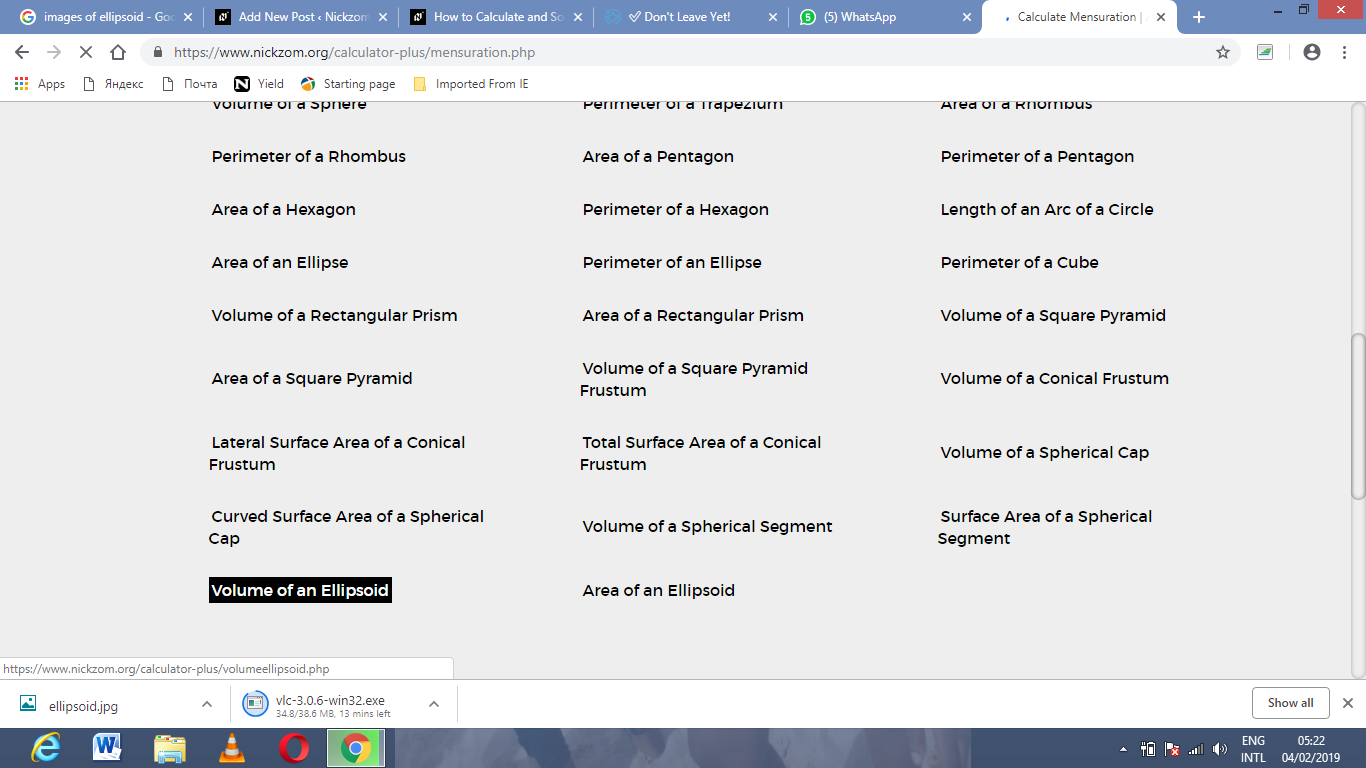# How to Calculate and Solve for the Axis and Volume of an Ellipsoid | The Calculator EncyclopediaThe image above is an ellipsoid.

To compute the volume of an ellipsoid, three essential parameters are needed and this parameters are axis (a)axis (b) and axis (c).

The formula for calculating the volume of an ellipsoid:

V = 4πabc3

Where;

V = Volume of the ellipsoid
a = Axis of the ellipsoid
b = Axis of the ellipsoid
c = Axis of the ellipsoid

Let’s solve an example;
Given that the axis of the ellipsoid (a) is 9 cm and axis of the ellipsoid (b) is 11 cm with the axis (c) of 14 cm. Find the volume of the ellipsoid?

This implies that;
a = Axis of the ellipsoid = 9 cm
b = Axis of the ellipsoid = 11 cm
c = Axis of the ellipsoid = 14 cm

V = 4πabc3
V = 4π(9 x 11 x 14)3
V = 4π(1386)3
V = (12.56)(1386) / 3
V = (17416.98967) / 3
V = 5805.66

Therefore, the volume of the ellipsoid is 5805.66 cm3.

Calculating the Axis (a) of an Ellipsoid using the Volume of the Ellipsoid, Axis (b) of the Ellipsoid and Axis (c) of the Ellipsoid.

a = V3 / 4πbc

Where;
a = Axis of the ellipsoid
V = Volume of the ellipsoid
b = Axis of the ellipsoid
c = Axis of the ellipsoid

Let’s solve an example;
Find the axis (a) of an ellipsoid when the volume of the ellipsoid is 280 cm3 with an axis (b) of 18 cm and axis (c) of 8 cm.

This implies that;
V = Volume of the ellipsoid = 280 cm3
b = Axis of the ellipsoid = 18 cm
c = Axis of the ellipsoid = 8 cm

a = V3 / 4πbc
a = 280 x 3 / (12.566)(18 x 8)
a = 840 / (12.566)(144)
a = 840 / 1809.504
a = 0.46

Therefore, the axis (a) of the ellipsoid is 0.46 cm.

Calculating the Axis (b) of an Ellipsoid using the Volume of the Ellipsoid, Axis (a) of the Ellipsoid and Axis (c) of the Ellipsoid.

b = V3 / 4πac

Where;
b = Axis of the ellipsoid
V = Volume of the ellipsoid
a = Axis of the ellipsoid
c = Axis of the ellipsoid

Let’s solve an example;
Find the axis (b) of an ellipsoid when the volume of the ellipsoid is 320 cm3 with an axis (a) of 19 cm and axis (c) of 7 cm.

This implies that;
V = Volume of the ellipsoid = 320 cm3
a = Axis of the ellipsoid = 19 cm
c = Axis of the ellipsoid = 7 cm

b = V3 / 4πac
b = 320 x 3 / (12.566)(19 x 7)
b = 960 / (12.566)(133)
b = 960 / 1671.278
b = 0.574

Therefore, the axis (b) of the ellipsoid is 0.574 cm.

Calculating the Axis (c) of an Ellipsoid using the Volume of the Ellipsoid, Axis (a) of the Ellipsoid and Axis (b) of the Ellipsoid.

c = V3 / 4πab

Where;
c = Axis of the ellipsoid
V = Volume of the ellipsoid
a = Axis of the ellipsoid
b = Axis of the ellipsoid

Let’s solve an example;
Find the axis (c) of an ellipsoid when the volume of the ellipsoid is 480 cm3 with an axis (a) of 22 cm and axis (b) of 11 cm.

This implies that;
V = Volume of the ellipsoid = 480 cm3
a = Axis of the ellipsoid = 22 cm
b = Axis of the ellipsoid = 11 cm

c = V3 / 4πab
c = 480 x 3 / (12.566)(22 x 11)
c = 1440 / (12.566)(242)
c = 1440 / 3040.972
c = 0.474

Therefore, the axis (c) of the ellipsoid is 0.474 cm.

Nickzom Calculator – The Calculator Encyclopedia is capable of calculating the volume of an ellipsoid.

To get the answer and workings of the volume of an ellipsoid using the Nickzom Calculator – The Calculator Encyclopedia. First, you need to obtain the app.

You can get this app via any of these means:

To get access to the professional version via web, you need to register and subscribe for NGN 1,500 per annum to have utter access to all functionalities.
You can also try the demo version via https://www.nickzom.org/calculator

Once, you have obtained the calculator encyclopedia app, proceed to the Calculator Map, then click on Mensuration under the Mathematics sectionNow, click on Volume of an Ellipsoid under MensurationThe screenshot below displays the page or activity to enter your values, to get the answer for the volume of an ellipsoid according to the respective parameters which are the axis (a)axis (b) and axis (c) of the ellipsoid.Now, enter the values appropriately and accordingly for the parameters as required by the example above where the axis (a) is 9 cmaxis (b) is 11 cm and  axis (c) is  14 cm.Finally, Click on CalculateAs you can see from the screenshot above, Nickzom Calculator – The Calculator Encyclopedia solves for the volume of the ellipsoid and presents the formula, workings and steps too.